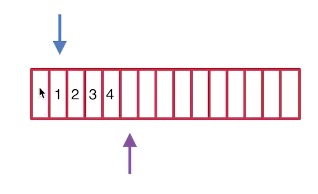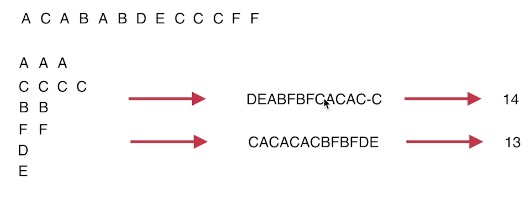# JS基础算法(数据结构之队列篇)

• 前端
• 面试
• 算法原创

## 数据结构之队列

### 设计循环队列

• MyCircularQueue(k): 构造器，设置队列长度为 k 。
• Front: 从队首获取元素。如果队列为空，返回 -1 。
• Rear: 获取队尾元素。如果队列为空，返回 -1 。
• enQueue(value): 向循环队列插入一个元素。如果成功插入则返回真。
• deQueue(): 从循环队列中删除一个元素。如果成功删除则返回真。
• isEmpty(): 检查循环队列是否为空。
• isFull(): 检查循环队列是否已满。

#### 示例：

MyCircularQueue circularQueue = new MycircularQueue(3); // 设置长度为 3

circularQueue.enQueue(1); // 返回 true

circularQueue.enQueue(2); // 返回 true

circularQueue.enQueue(3); // 返回 true

circularQueue.enQueue(4); // 返回 false，队列已满

circularQueue.Rear(); // 返回 3

circularQueue.isFull(); // 返回 true

circularQueue.deQueue(); // 返回 true

circularQueue.enQueue(4); // 返回 true

circularQueue.Rear(); // 返回 4export default class MyCircularQueue {
constructor (k) {
// 保存数据长度为k的数据结构
this.list=Array(k)
// 队首的指针
this.front=0
// 队尾的指针
this.rear=0
// 队列的长度
this.max=k
}
enQueue (num) {
if(this.isFull()){
return false
}else{
this.list[this.rear]=num
this.rear=(this.rear+1)%this.max
return true
}
}
deQueue () {
let v=this.list[this.front]
this.list[this.front]=''
this.front=(this.front+1)%this.max
return v
}
isEmpty () {
return this.front===this.rear&&!this.list[this.front]
}
isFull () {
return this.front===this.rear&&!!this.list[this.front]
}
Front () {
return this.list[this.front]
}
Rear () {
let rear=this.rear-1

return this.list[rear<0?this.max-1:rear]
}
}

class MycircularQueue{
constructor(len){
this.list=new Array(len);
this.front=0;
this.rear=0;
this.max=len;
}
Front(){
return this.list.length?this.list(this.front):-1;
}
Rear(){
let rear=this.rear-1;
return this.list[rear<0?this.max-1:rear]
}
enQueue(value){
if(this.isFull()){
return false;
}else{
this.list[this.rear]=value;
this.rear=(this.rear+1)%this.max;
return true;
}
}
deQueue(){
if(this.list.length>0){
this.list[this.front]="";
this.front++;
return true;
}else{
return false;
}
}
isEmpty(){
return this.front===this.rear&&!this.list[this.front]
}
isFull(){
return this.front===this.rear&&this.list[this.front]
}
}


### 任务调度器

#### 示例 1：

n 的取值范围为 [0, 100]。export default (tasks, n) => {
let q = ''
let Q = {}
// 每种任务的数量
if (Q[item]) {
Q[item]++
} else {
Q[item] = 1
}
})
while (1) {
let keys = Object.keys(Q)
if (!keys) {
break
}
// n+1为一组
let tmp = []
for (let i = 0; i <= n; i++) {
let max = 0
let key
let pos
// 从所有的任务中找到未处理数最大的，优先安排
keys.forEach((item, idx) => {
if (Q[item] > max) {
max = Q[item]
key = item
pos = idx
}
})
if (key) {
tmp.push(key)
keys.splice(pos, 1)
Q[key]--;
if (Q[key] < 1) {
delete Q[key]
}
} else {
break
}
}
q += tmp.join('').padEnd(n + 1, '-')
}
// A--A--A--
q = q.replace(/-+\$/g, '')
return q.length
}


0

0

0